##### Actions

A linear operator(where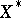and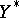are the strong duals of locally convex spacesand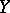, respectively), constructed from a linear operatorin the following way. Let the domain of definition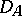ofbe everywhere dense in. If for all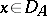,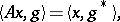(*)
where,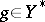and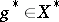, then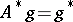is a uniquely defined operator from the set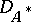of elementssatisfying (*) into. If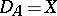andis continuous, then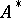is also continuous. If, in addition,andare normed linear spaces, then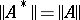. Ifis completely continuous, then so is. Adjoint operators are of particular interest in the case whenandare Hilbert spaces.